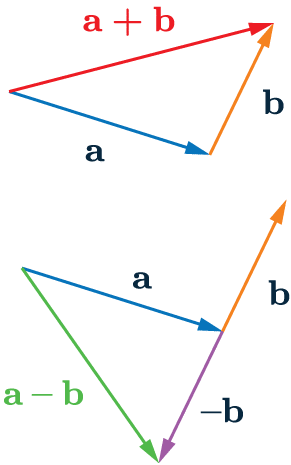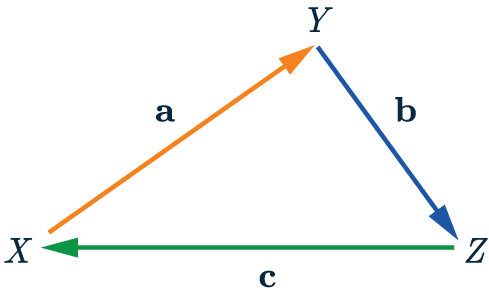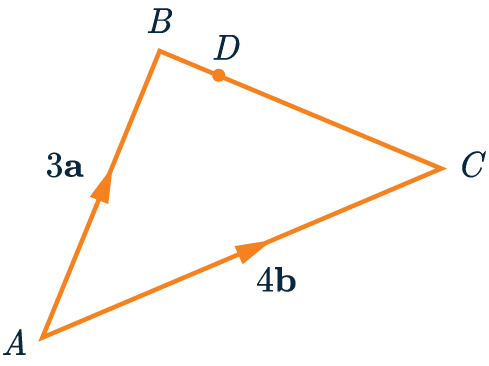# Vectors

GCSELevel 6-7Level 8-9AQAEdexcelOCR

## Vectors

A vector is something with both magnitude and direction. On diagrams they are denoted by an arrow, where the length tells us the magnitude and the arrow tells us direction.

• You will need to add and subtract vectors
• You will also need to multiply vectors and understand scalar multiples of vectorsMake sure you are happy with the following topics before continuing:

Level 6-7GCSEAQAEdexcelOCR

$\textcolor{red}{\mathbf{a}+\mathbf{b}}$

this takes you from the start of $\mathbf{a}$ to the end of $\mathbf{b}$ (right).

The negative of a vector has the same magnitude of the original vector, it just goes in the exact opposite direction.

When we subtract vectors:

$\textcolor{limegreen}{\mathbf{a}-\mathbf{b}}$,

we add on the negative of the vector that is being subtracted.Level 6-7GCSEAQAEdexcelOCR

## Multiplying Vectors

Multiplying vectors by a Scalar – We can  multiply a vector by a number. For example, we can multiply $\mathbf{a}$ by $3$:

$3\mathbf{\textcolor{red}{a}}=\mathbf{\textcolor{red}{a}}+\mathbf{\textcolor{red}{a}}+\mathbf{\textcolor{red}{a}}$

This means the the vectors are added end to end.

We can multiply more complicated vectors

$3\mathbf{(\textcolor{red}{a}+\textcolor{blue}{b})}=\mathbf{3\textcolor{red}{a}}+\mathbf{3\textcolor{blue}{b}}$

Note: all vectors here are written in bold. When you’re writing this by hand, you should underline each letter that represents a vector.

Scalar multiples – Scalar multiples are all parallel to each other:

$\mathbf{3\textcolor{red}{a}}+\mathbf{3\textcolor{blue}{b}}$ is parallel to $\mathbf{\textcolor{red}{a}}+\mathbf{\textcolor{blue}{b}}$

Level 6-7GCSEAQAEdexcelOCR

## Vector Notation

A vector from point $X$ to point $Y$ will be shown as $\overrightarrow{XY}$.So from the diagram shown we can see that:

$\overrightarrow{XY} = \mathbf{a}$

$\overrightarrow{YZ} = \mathbf{b}$

$\overrightarrow{ZX} = \mathbf{c}$

If we go against the arrow the vector becomes negative.

$\overrightarrow{YX} = \mathbf{-a}$

We can also combine vectors, meaning

$\overrightarrow{XZ} = \mathbf{a + b} = \mathbf{-c}$

Level 6-7GCSEAQAEdexcelOCR
Level 6-7GCSEAQAEdexcelOCR

## Example: Vectors and Ratios

In the diagram below, we have vectors

$\overrightarrow{AB}=3\mathbf{a}$ and $\overrightarrow{AC}=4\mathbf{b}$ .

Point $D$ lies on the  line $BC$ such that $BD:DC=1:3$.

Write the vector $\overrightarrow{AD}$ in terms of $\mathbf{a}$ and $\mathbf{b}$.

[3 marks]To find $\overrightarrow{AD}$, we are going to add the vectors

$\overrightarrow{AB}+\overrightarrow{BD}$

We know $\overrightarrow{AB}=3\mathbf{a}$ but we need to find $\overrightarrow{BD}$

We know $BD:DC=1:3$, so the point $D$ is $\dfrac{1}{4}$ of the way along $\overrightarrow{BC}$

$\overrightarrow{BC}=-\overrightarrow{AB}+\overrightarrow{AC}=-3\mathbf{a}+4\mathbf{b}$

Now we need to multiply this by $\dfrac{1}{4}$

$\overrightarrow{BD}=\dfrac{1}{4}(3\mathbf{a}+4\mathbf{b})=-\dfrac{3}{4}\mathbf{a}+\mathbf{b}$

Now we need to add  $\overrightarrow{AB}$ to the answer:

$\overrightarrow{AD}=\overrightarrow{AB}+\overrightarrow{BD}=3\mathbf{a}+\left(-\dfrac{3}{4}\mathbf{a}+\mathbf{b}\right)=\dfrac{9}{4}\mathbf{a}+\mathbf{b}$

Thus, we have our answer in terms of $\mathbf{a}$ and $\mathbf{b}$.

Level 8-9GCSEAQAEdexcelOCR

## Vectors Example Questions

First we can find the vector $\overrightarrow{AC}$

$\overrightarrow{AC} =\overrightarrow{AB} +\overrightarrow{BC} =3\mathbf{a} +2\mathbf{b}$

Since $M$ is the midpoint of $AC$ we know that $\overrightarrow{AM}=\dfrac{1}{2}\overrightarrow{AC}$

$\overrightarrow{AM}=\dfrac{1}{2}(3\mathbf{a} +2\mathbf{b}) = \dfrac{3}{2}\mathbf{a} +\mathbf{b}$Gold Standard Education

The vector $\overrightarrow{EF}$ is the same as:

$\overrightarrow{EF}=\overrightarrow{ED}+\overrightarrow{DC}+\overrightarrow{CF}$

First, we can find the vector $\overrightarrow{ED}$ as $\dfrac{1}{2}\mathbf{b}$.

Then we also know $\overrightarrow{DC} = \overrightarrow{AB}$, hence $\overrightarrow{DC}=2\mathbf{a}$.

The final section $\overrightarrow{CF} = \dfrac{3}{5}\times 2\mathbf{a}=\dfrac{6}{5}\mathbf{a}$

Hence,

$\overrightarrow{EF}= \dfrac{1}{2} \mathbf{b}+2\mathbf{a}+\dfrac{6}{5}\mathbf{a}=3.2\mathbf{a}+ \dfrac{1}{2} \mathbf{b}$Gold Standard Education

First we can find the vector $\overrightarrow{BC}$

$\overrightarrow{BC} =-\overrightarrow{AB} +\overrightarrow{AC} =-\mathbf{a} +2\mathbf{b}$

Then we can find:

$\overrightarrow{BD} =\dfrac{3}{5}\overrightarrow{BC} =-\dfrac{3}{5}\mathbf{a} +\dfrac{6}{5}\mathbf{b}$

Next:

\begin{aligned} \overrightarrow{AD} &=\overrightarrow{AB}+\overrightarrow{BD} \\ &=\mathbf{a} +\bigg(-\dfrac{3}{5}\mathbf{a} +\dfrac{6}{5}\mathbf{b}\bigg) \\ & =\dfrac{2}{5}\mathbf{a}+\dfrac{6}{5}\mathbf{b} \end{aligned}

Finally:

\begin{aligned} \overrightarrow{AE} &=\dfrac{1}{3}\overrightarrow{AD} \\ &=\dfrac{1}{3}\bigg(\dfrac{2}{5}\mathbf{a}+\dfrac{6}{5}\mathbf{b}\bigg) \\ &= \dfrac{2}{15}\mathbf{a}+\dfrac{2}{5}\mathbf{b} \end{aligned}Gold Standard Education

We will find $\overrightarrow{AC}$ by doing,

$\overrightarrow{AC}=\overrightarrow{AB}-\overrightarrow{OB}+\overrightarrow{OC}$

Thus

$\overrightarrow{AC} =\mathbf{b}-\mathbf{a}+\mathbf{b}= 2\mathbf{b}-\mathbf{a}$Gold Standard Education

We will find $\overrightarrow{EB}$ by doing

$\overrightarrow{EB}=\overrightarrow{EA}+\overrightarrow{AB}$

The first vector is straightforward, because we know $\overrightarrow{AE}$, and that is just the same vector in the opposite direction. So, we get:

$\overrightarrow{EA}=-\overrightarrow{AE}=-(3\mathbf{a}-2\mathbf{b})=-3\mathbf{a}+2\mathbf{b}$

Now we need $\overrightarrow{AB}$. Since $B$ is the midpoint of $AC$ (given in the question), we must have that $\overrightarrow{AB}=\frac{1}{2} \overrightarrow{AC}$. Therefore, looking at the diagram, we get that:

$\overrightarrow{AB}=\dfrac{1}{2}\overrightarrow{AC}=\dfrac{1}{2}\left(\overrightarrow{AD}+\overrightarrow{DC}\right)$

We’re given the second part of this, $\overrightarrow{DC}=2\mathbf{a}+4\mathbf{b}$, and since $E$ is the midpoint of $AD$, we can also work out the first part:

$\overrightarrow{AD}=2\overrightarrow{AE}=2(3\mathbf{a}-2\mathbf{b})=6\mathbf{a}-4\mathbf{b}$

Now, we have everything we need and can go back through our work, filling in the gaps we find:

$\overrightarrow{AB}=\dfrac{1}{2}\left(6\mathbf{a}-4\mathbf{b}+2\mathbf{a} +4\mathbf{b}\right)=\dfrac{1}{2}\left(8\mathbf{a}\right)=4\mathbf{a}$

Finally we have that:

$\overrightarrow{EB}=\overrightarrow{EA}+\overrightarrow{AB}=-3\mathbf{a}+2\mathbf{b}+4\mathbf{a}=\mathbf{a}+2\mathbf{b}$

If $\overrightarrow{EB}$ and $\overrightarrow{DC}$ are parallel, then one must be a multiple of the other. Well, if we multiply $\overrightarrow{EB}$ by 2 then,

$2\times\overrightarrow{EB}=2(\mathbf{a}+2\mathbf{b})=2\mathbf{a}+4\mathbf{b}=\overrightarrow{DC}$

We have shown that $2\overrightarrow{EB}=\overrightarrow{DC}$, therefore, the two lines must be parallel.Gold Standard Education

## Vectors Worksheet and Example Questions

### (NEW) Vectors Exam Style Questions - MME

Level 6-7GCSENewOfficial MMEGold Standard Education

Level 4-5GCSE